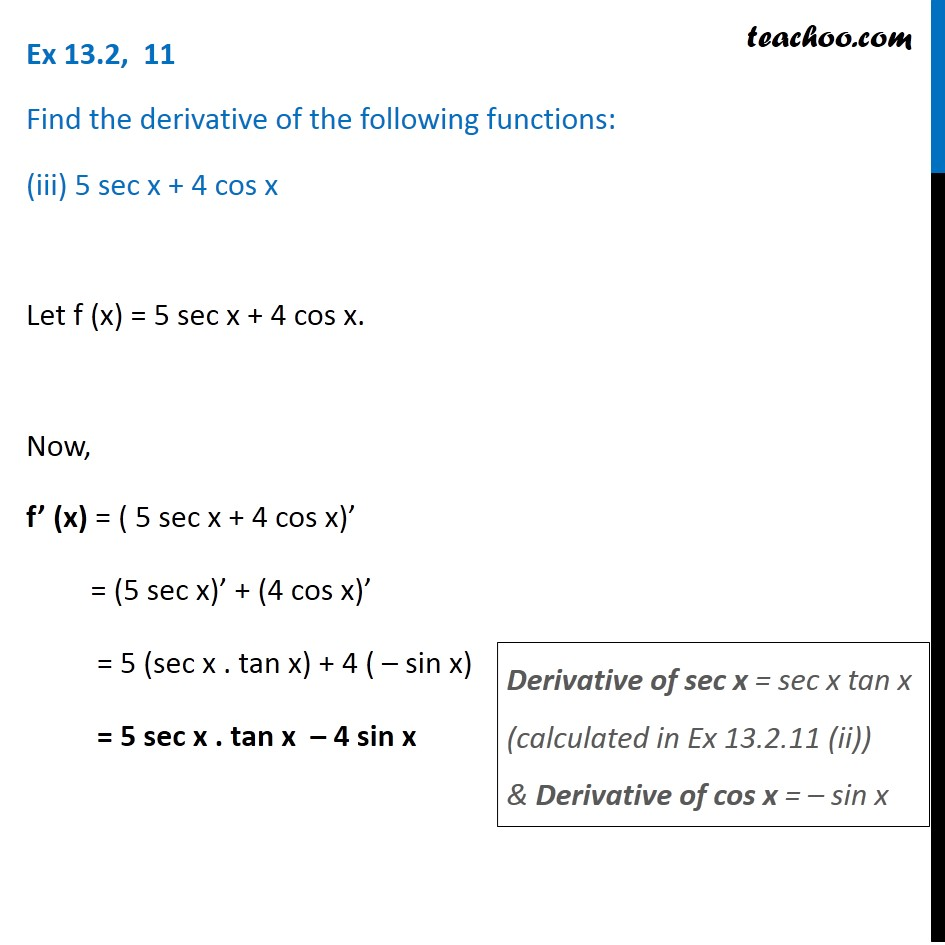Ex 12.2

Chapter 12 Class 11 Limits and Derivatives
Serial order wiseLearn in your speed, with individual attention - Teachoo Maths 1-on-1 Class

### Transcript

Ex 12.2, 11 Find the derivative of the following functions: (iii) 5 sec x + 4 cos x Let f (x) = 5 sec x + 4 cos x. Now, f’ (x) = ( 5 sec x + 4 cos x)’ = (5 sec x)’ + (4 cos x)’ = 5 (sec x . tan x) + 4 ( – sin x) = 5 sec x . tan x – 4 sin x Derivative of sec x = sec x tan x (calculated in Ex 12.2.11 (ii)) & Derivative of cos x = – sin x